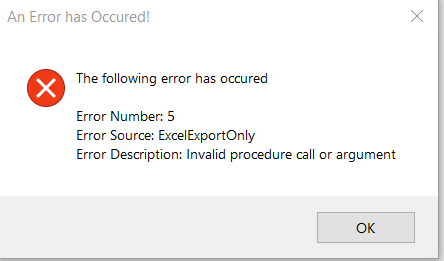troubleshooting Question

# Access VBA - Error when exporting to Excelasked on
Microsoft AccessVBA
Hi

I am getting the following error when running the code below to export data. It happens at the line "  Worksheet.Range("A2").CopyFromRecordset CRecordset"
The spreadsheet is still created though Book1.xlsx``````Public Function ExcelExportAndFormat(ByVal CRecordset As DAO.Recordset, ByVal CSheetName As String) As Object

On Error GoTo Error_Handler

Dim Excel As Object ' Excel.Application
Dim Workbook As Object ' Excel.Workbook
Dim Worksheet As Object ' Excel.Worksheet

Dim Count As Long

Set ExcelExportAndFormat = Nothing
Set Excel = CreateObject("Excel.Application")

Set Worksheet = Workbook.Sheets(1)
Worksheet.Name = CSheetName
For Count = 0 To CRecordset.Fields.Count - 1
Worksheet.Range("A1").Offset(, Count).Value = CStr(CRecordset.Fields(Count).Name)
Next Count

'//NOTE: The following line is highly important to stop green error messages
Excel.Application.ErrorCheckingOptions.NumberAsText = False '// stop number store as text error

Worksheet.Range("A2").CopyFromRecordset CRecordset

Worksheet.Rows(1).Font.Bold = True
Excel.Rows("2:2").Select
Excel.ActiveWindow.FreezePanes = True

If blnExcelValidationsTable = True Then
Excel.Cells.RowHeight = 15
Excel.Columns("A:A").ColumnWidth = 3.89
Excel.Columns("B:B").ColumnWidth = 74.89
Excel.Columns("C:C").ColumnWidth = 74.89
Excel.Columns("D:D").ColumnWidth = 27.89
Excel.Columns("E:E").ColumnWidth = 69.89
Excel.ActiveWindow.DisplayGridlines = True
Else
Worksheet.Cells.EntireColumn.AutoFit
Excel.ActiveWindow.DisplayGridlines = False
Excel.Rows("1:1").RowHeight = 21.6
End If

'// take care of green "numbers stored as text" error [This did not work!]
''Dim c As Variant
''Dim sUsedRange As String: sUsedRange = Worksheet.UsedRange.Address
'MsgBox sUsedRange
''Worksheet.Activate 'Without this the next few lines don't work..
''For Each c In Worksheet.UsedRange.Cells
''c.Errors(xlNumberAsText).Ignore = True
''Next
''For Each c In Excel.ActiveSheet.UsedRange.Cells
''c.Errors(xlNumberAsText).Ignore = True
''Next
''//

Set ExcelExportAndFormat = Worksheet
Excel.Visible = True
Set Worksheet = Nothing
Set Workbook = Nothing
Set Excel = Nothing

Error_Handler_Exit:
On Error Resume Next
Excel.Visible = True
If Not Worksheet Is Nothing Then Set Worksheet = Nothing
If Not Workbook Is Nothing Then Set Workbook = Nothing
If Not Excel Is Nothing Then Set Excel = Nothing
Exit Function

Error_Handler:

MsgBox "The following error has occured" & vbCrLf & vbCrLf & _
"Error Number: " & Err.Number & vbCrLf & _
"Error Source: ExcelExportOnly" & vbCrLf & _
"Error Description: " & Err.Description & _
Switch(Erl = 0, "", Erl <> 0, vbCrLf & "Line No: " & Erl) _
, vbOKOnly + vbCritical, "An Error has Occured!"

Resume Error_Handler_Exit

End Function
``````
ste5an
Senior Developer
###### Learn from the best

Network and collaborate with thousands of CTOs, CISOs, and IT Pros rooting for you and your success.

Andrew Hancock - VMware vExpert
See if this solution works for you by signing up for a 7 day free trial.